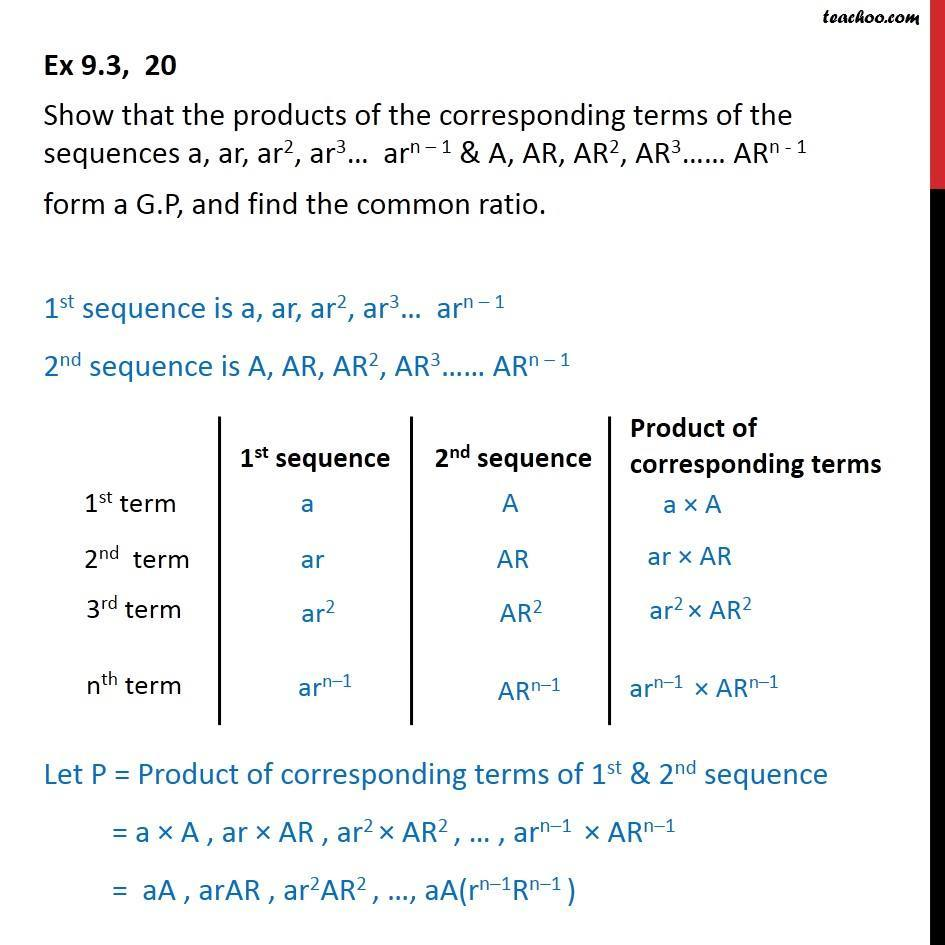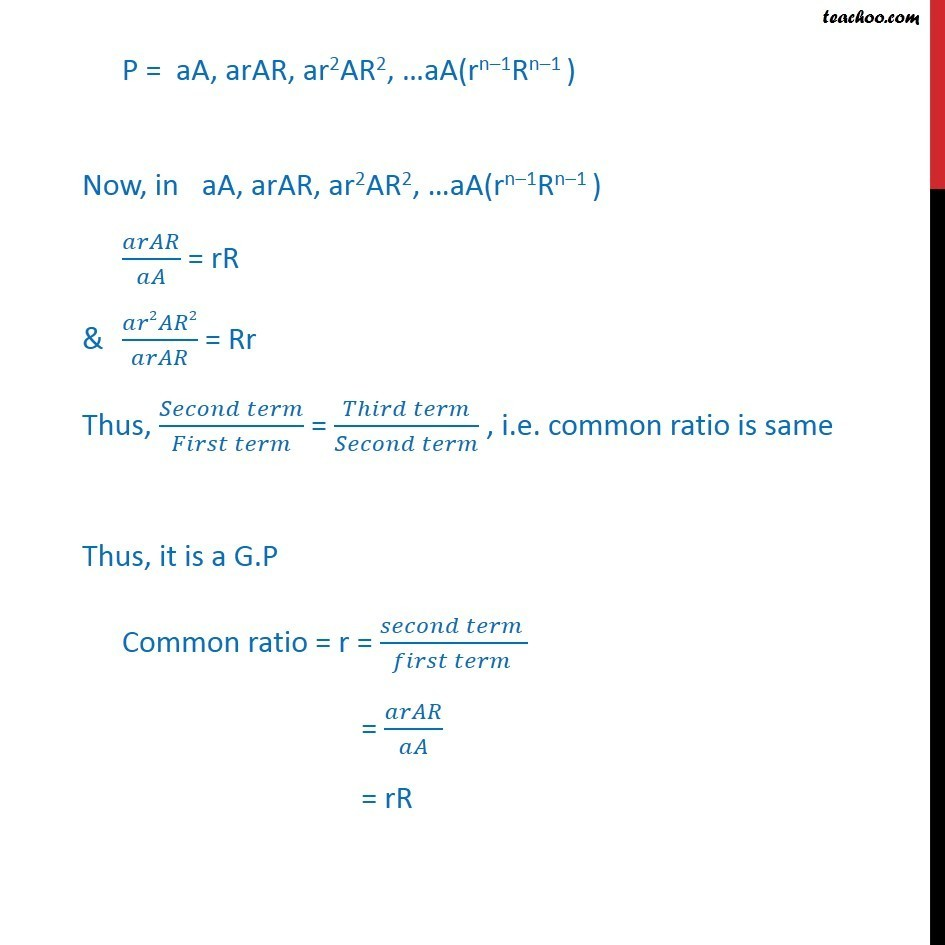Geometric Progression(GP): Formulae based

Chapter 8 Class 11 Sequences and Series
Concept wiseLearn in your speed, with individual attention - Teachoo Maths 1-on-1 Class

### Transcript

Ex 8.2, 20 Show that the products of the corresponding terms of the sequences a, ar, ar2, ar3… arn – 1 & A, AR, AR2, AR3…… ARn - 1 form a G.P, and find the common ratio. 1st sequence is a, ar, ar2, ar3… arn – 1 2nd sequence is A, AR, AR2, AR3…… ARn – 1 Let P = Product of corresponding terms of 1st & 2nd sequence = a × A , ar × AR , ar2 × AR2 , … , arn–1 × ARn–1 = aA , arAR , ar2AR2 , …, aA(rn–1Rn–1 ) P = aA, arAR, ar2AR2, …aA(rn–1Rn–1 ) Now, in aA, arAR, ar2AR2, …aA(rn–1Rn–1 ) 𝑎𝑟𝐴𝑅/𝑎𝐴 = rR & 𝑎𝑟2𝐴𝑅2/𝑎𝑟𝐴𝑅 = Rr Thus, (𝑆𝑒𝑐𝑜𝑛𝑑 𝑡𝑒𝑟𝑚)/(𝐹𝑖𝑟𝑠𝑡 𝑡𝑒𝑟𝑚) = (𝑇ℎ𝑖𝑟𝑑 𝑡𝑒𝑟𝑚)/(𝑆𝑒𝑐𝑜𝑛𝑑 𝑡𝑒𝑟𝑚) , i.e. common ratio is same Thus, it is a G.P Common ratio = r = (𝑠𝑒𝑐𝑜𝑛𝑑 𝑡𝑒𝑟𝑚 )/(𝑓𝑖𝑟𝑠𝑡 𝑡𝑒𝑟𝑚 ) = 𝑎𝑟𝐴𝑅/𝑎𝐴 = rR